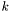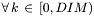# Point Class Reference

#include <Point.H>

## Detailed Description

A point in space.

An Integer valued point in space of arbitrary dimension. May also be used to represent a vector with integer components.

## Public Member Functions

Point ()
Default Constructor.
Point (const int a_tuple[DIM])
Construct a Point using an int array.
Point (const array< int, DIM > a_tuple)
Construct a Point using an int array.
Point (const Point &a_pt)
Copy Constructor.
bool operator< (const Point &a_rhs) const
Definition of "<" operator on Points. Returns true if this* is "less than" *a_rhs.
Point operator+ (const Point &a_rhsPoint) const
Point operator- (const Point &a_rhsPoint) const
Componetwise subtraction of two Points.
Point operator/ (int a_nref) const
Componentwise division of a Point by an Integer.
Point operator/ (const Point &a_pt) const
Componentwise division of a Point by another Point.
Point operator* (int a_nref) const
Componentwise multiplication of a Point by an Integer.
Point operator* (const Point &a_pt) const
Componentwise multiplication between two Points.
void operator*= (const Point &a_pt)
Multiplication of two Points in place.
void operator+= (const Point &a_pt)
Addition of two Points in place.
void operator-= (const Point &a_pt)
Subtraction of two Points in place.
void operator/= (const Point &a_pt)
Division of two Points in place.
void operator*= (int a_n)
Multiplication in place by an Integer.
void operator+= (int a_n)
Addition in place by an Integer.
void operator-= (int a_n)
Subtraction in place by an Integer.
void operator/= (int a_n)
Division in place by an Integer.
bool operator== (const Point &a_pt) const
Equality between two Points.
bool operator!= (const Point &a_pt) const
Inequality between two Points.
const int & operator[] (const int &a_index) const
Get component of *this corresponding to a_index.
int & operator[] (const int &a_index)
Get component of *this corresponding to a_index.
void print () const
Print out the contents of a Point.
void print2 () const
Print out the contents of a Point.

## Private Attributes

int m_tuple [DIM]
Integer coordinates of the Point.

## Constructor & Destructor Documentation

 Point::Point ( )  [inline]

Default Constructor.

 Point::Point ( const int a_tuple[DIM] )  [inline]

Construct a Point using an int array.

References m_tuple.

 Point::Point ( const array< int, DIM > a_tuple )  [inline]

Construct a Point using an int array.

References m_tuple.

 Point::Point ( const Point & a_pt )  [inline]

Copy Constructor.

## Member Function Documentation

 bool Point::operator< ( const Point & a_rhs ) const [inline]

Definition of "<" operator on Points. Returns true if this* is "less than" *a_rhs.

This is just an ordering. It is used to add Points to maps etc. Don't use it to actually compare Points.

This is just an ordering. It is used to add Points to maps etc. Don't use it to actually compare Points.

References m_tuple.

 Point Point::operator+ ( const Point & a_rhsPoint ) const [inline]

References m_tuple.

 Point Point::operator- ( const Point & a_rhsPoint ) const [inline]

Componetwise subtraction of two Points.

References m_tuple.

 Point Point::operator/ ( int a_nref ) const [inline]

Componentwise division of a Point by an Integer.

Quotients are rounded down. Division of any component by 0 yields an error.

References m_tuple.

 Point Point::operator/ ( const Point & a_pt ) const [inline]

Componentwise division of a Point by another Point.

Quotients are rounded down. Division of any component by 0 yields an error.

References m_tuple.

 Point Point::operator* ( int a_nref ) const [inline]

Componentwise multiplication of a Point by an Integer.

References m_tuple.

 Point Point::operator* ( const Point & a_pt ) const [inline]

Componentwise multiplication between two Points.

References m_tuple.

 void Point::operator*= ( const Point & a_pt )  [inline]

Multiplication of two Points in place.

References m_tuple.

 void Point::operator+= ( const Point & a_pt )  [inline]

Addition of two Points in place.

References m_tuple.

 void Point::operator-= ( const Point & a_pt )  [inline]

Subtraction of two Points in place.

References m_tuple.

 void Point::operator/= ( const Point & a_pt )  [inline]

Division of two Points in place.

Quotients are rounded down. Division of any component by 0 yields an error.

References m_tuple.

 void Point::operator*= ( int a_n )  [inline]

Multiplication in place by an Integer.

References m_tuple.

 void Point::operator+= ( int a_n )  [inline]

Addition in place by an Integer.

References m_tuple.

 void Point::operator-= ( int a_n )  [inline]

Subtraction in place by an Integer.

References m_tuple.

 void Point::operator/= ( int a_n )  [inline]

Division in place by an Integer.

Quotients are rounded down. Division of any component by 0 yields an error.

References m_tuple.

 bool Point::operator== ( const Point & a_pt ) const [inline]

Equality between two Points.

Returns true if *this[] == *a_pt[]References m_tuple.

 bool Point::operator!= ( const Point & a_pt ) const [inline]

Inequality between two Points.

Returns false if *this == *a_pt.

 const int& Point::operator[] ( const int & a_index ) const [inline]

Get component of *this corresponding to a_index.

For use with constant Points

References m_tuple.

 int& Point::operator[] ( const int & a_index )  [inline]

Get component of *this corresponding to a_index.

References m_tuple.

 void Point::print ( ) const [inline]

Print out the contents of a Point.

Output is formatted: (p1,p2,...)

References m_tuple.

Referenced by Stencil< T >::print(), and Shift::print().

 void Point::print2 ( ) const [inline]

Print out the contents of a Point.

Output is formatted: p1 p2 p3 ...

References m_tuple.

## Member Data Documentation

 int Point::m_tuple[DIM] [private]

Integer coordinates of the Point.

The documentation for this class was generated from the following files:

Generated on Fri Mar 11 12:53:44 2016 for AMRStencil by1.5.5